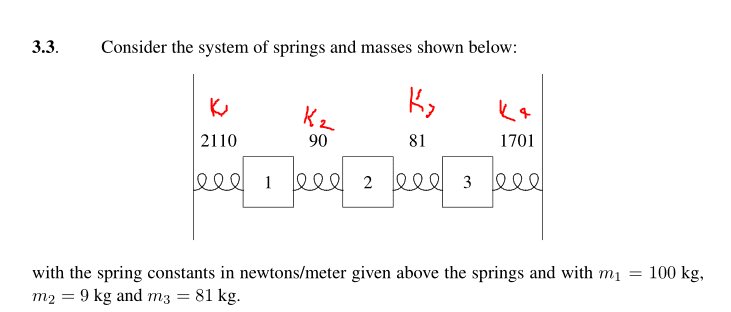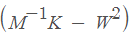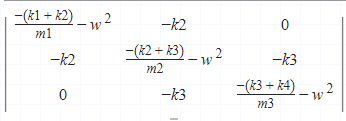# Spring constant matrix and normal modes (4 springs and 3 masses)

LCSphysicist
Homework Statement:
ALl below
Relevant Equations:
ALl below
We need to find the normal modes of this system:Well, this system is a little easy to deal when we put it in a system and solve the system... That's not what i want to do, i want to try my direct matrix methods.

We have springs with stiffness k1,k2,k3,k4 respectively, and block mass m1, m2, m3
And we need to annul the determinant of, not just, i supposed the displacement Z as complex, so we need real angular frequencies.

To mount the matrix K, we need to know all Kij, being Kij the spring stiffness equivalent if we move just block j and analyze the motion of block i, j can be equal i.

So making all the things, we end with:Apparently this is wrong, i found just complex w, what is the problem?

#### Attachments

•